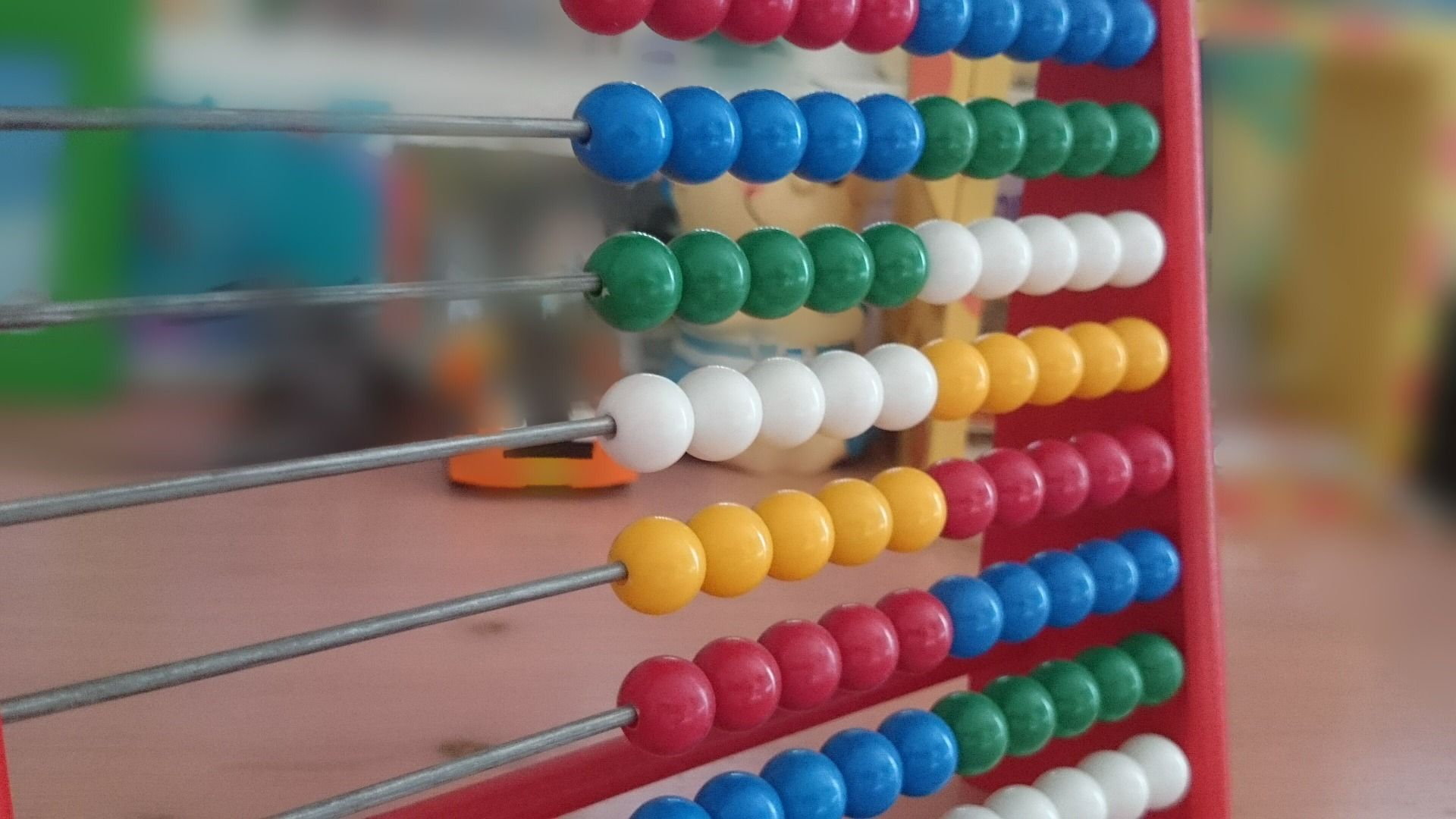This lesson was designed to be a fun approach to using the abacus for place value understanding and properties of operations to add and subtract.

This activity will also help students develop:

• Knowledge of place value (tens and one’s place)
• Use of the abacus for direct/indirect addition and subtraction
• Familiarity with tactile dice
• Abacus
• Tactile dice (2 per player)
• Base 10 set: ten rods, unit cubes
1. Each student rolls one dice at a time and sets that number in the tens place on the abacus.
2. Next the student rolls the second dice and sets that number in the ones place on the abacus.
3. Once the two-digit number is set on the abacus, students are asked to use manipulatives (unit cubes, ten rods) to represent their two-digit number.
4. After each student has correctly displayed their number they can compare numbers. The student with the largest number wins that round.

Extension: Students can practice addition/subtraction skills on the abacus by rolling one dice again and adding/subtracting that number to the original number set on the abacus.

• Use a piece of felt on the abacus to cover columns not being used (thousands place and higher)
• Use a sorting tray to separate ten rods and unit cubes
• If the game is being played with sighted peers they can use paper/pencil to record their numbers

### Domain Math:

Number & Operations in Base Ten

### Math Standard:

• 1.NBT.B.2a – Understand place value. – Understand that the two digits of a two-digit number represent amounts of tens and ones. Understand the following as a special case: 10 can be thought of as a bundle of ten ones — called a “ten.”
• 1.NBT.B.2b – Understand place value. – Understand that the two digits of a two-digit number represent amounts of tens and ones. Understand the following as a special case: The numbers from 11 to 19 are composed of a ten and one, two, three, four, fiv…
• 1.NBT.B.2c – Understand place value. – Understand that the two digits of a two-digit number represent amounts of tens and ones. Understand the following as a special case: The numbers 10, 20, 30, 40, 50, 60, 70, 80, 90 refer to one, two, three, four, …
• 1.NBT.C.4 – Use place value understanding and properties of operations to add and subtract. – Add within 100, including adding a two-digit number and a one-digit number, and adding a two-digit number and a multiple of 10, using concrete models or draw…